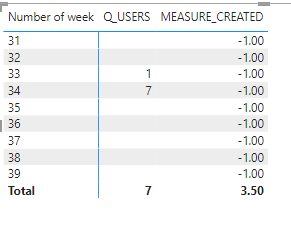cancel
Showing results for
Did you mean:

Fabric is Generally Available. Browse Fabric Presentations. Work towards your Fabric certification with the Cloud Skills Challenge.Frequent Visitor

## DISTINCTCOUNT AT WEEK LEVEL

Hi! I hope you are well. I was developing a Power BI measure and I found a problem:
I want to see variation of clients that enter to an app per week.

I have a table like these in which "Alta_Pedido_Dt" is the date in which the client entered the app, "Cliente" is the unique identifier of that client.What I expect is this:
The first two registers correspond to the first week and the same client, the other rows correspond to this week (7 different clients). I want to create a measure that does:
Measure = Quantity of different clients that entered the app this week(7) / Quantity of different clients that entered the last week(1) -1 = 6

I ve developed the next measures:
Q_USERS = DISTINCTCOUNT(TABLE1[Cliente])

USERS_VARIATION_PER_WEEK =
VAR _ACTUAL_WEEK = CALCULATE(SUMX(TABLE1,[Q_USERS]),FILTER(Calendar,Calendar[WEEK] = WEEKNUM(max(TABLE1[Alta_Pedido_Dt]),1)))

VAR _LAST_WEEK = CALCULATE(SUMX(TABLE1,[Q_USERS]),FILTER(Calendar,Calendar[WEEK] = (WEEKNUM(max(TABLE1[Alta_Pedido_Dt]),1)-1)))

RETURN
ROUND(DIVIDE(_ACTUAL_WEEK,_LAST_WEEK)-1,1)

But ive got an unsatisfying result:The value of the measure Q_USERS that i ve created is right for the week 33 and 34. But MEASURE_CREATED(USERS_VARIATION_PER_WEEK) is 3.5, why? There´s something that i am missing.

I wait for your help. Thank u so much!

1 ACCEPTED SOLUTIONSuper User

@TomasB , In the table use the Week from Date table, also Change measures like

and use the week rank as given below

Have these new columns in Date Table, Week Rank is Important in Date/Week Table

Week Rank = RANKX('Date','Date'[Week Start date],,ASC,Dense)
OR
Week Rank = RANKX('Date','Date'[Year Week],,ASC,Dense) //YYYYWW format

These measures can help
This Week = CALCULATE(sum('Table'[Qty]), FILTER(ALL('Date'),'Date'[Week Rank]=max('Date'[Week Rank])))
Last Week = CALCULATE(sum('Table'[Qty]), FILTER(ALL('Date'),'Date'[Week Rank]=max('Date'[Week Rank])-1))Super User

@TomasB , In the table use the Week from Date table, also Change measures like

and use the week rank as given below

Have these new columns in Date Table, Week Rank is Important in Date/Week Table

Week Rank = RANKX('Date','Date'[Week Start date],,ASC,Dense)
OR
Week Rank = RANKX('Date','Date'[Year Week],,ASC,Dense) //YYYYWW format

These measures can help
This Week = CALCULATE(sum('Table'[Qty]), FILTER(ALL('Date'),'Date'[Week Rank]=max('Date'[Week Rank])))
Last Week = CALCULATE(sum('Table'[Qty]), FILTER(ALL('Date'),'Date'[Week Rank]=max('Date'[Week Rank])-1))Announcements#### Power BI Monthly Update - November 2023

Check out the November 2023 Power BI update to learn about new features.#### Fabric Community News unified experience

Read the latest Fabric Community announcements, including updates on Power BI, Synapse, Data Factory and Data Activator.#### The largest Power BI and Fabric virtual conference

130+ sessions, 130+ speakers, Product managers, MVPs, and experts. All about Power BI and Fabric. Attend online or watch the recordings.Top Solution Authors
Top Kudoed Authors
Users online (2,195)